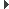SUAVE  2.5.2 An Aerospace Vehicle Environment for Designing Future AircraftSUAVE.Optimization.Package_Setups.TRMM.Trust_Region_Optimization.Trust_Region_Optimization Class Reference
Inheritance diagram for SUAVE.Optimization.Package_Setups.TRMM.Trust_Region_Optimization.Trust_Region_Optimization:## Public Member Functions

def __defaults__ (self)

def optimize (self, problem, print_output=False)

def evaluate_model (self, problem, x, der_flag=True)

def evaluate_corrected_model (self, x, problem=None, corrections=None, tr=None, return_cons=False)

def evaluate_constraints (self, x, problem=None, corrections=None, tr=None, lb=None, ub=None)

def calculate_constraint_violation (self, gval, lb, ub)

def calculate_correction (self, f, df, g, dg, tr)

def scale_vals (self, inp, con, ini, bnd, scl)

def accuracy_ratio (self, f_center, f_hi, f_corr, g_viol_center, g_viol_hi, g_viol_corr, tr)

def update_tr_size (self, rho, tr, accepted)

def initialize_SLSQP_constraints (self, con, problem, corrections, tr)

def unpack_constraints_slsqp (self, x, con_ind, sign, edge, problem, corrections, tr)Public Member Functions inherited from SUAVE.Core.Data.Data
def __getattribute__ (self, k)

def __setattr__ (self, k, v)

def __delattr__ (self, k)

def __new__ (cls, *args, **kwarg)

def typestring (self)

def dataname (self)

def __str__ (self, indent='')

def __init__ (self, *args, **kwarg)

def __iter__ (self)

def itervalues (self)

def values (self)

def update (self, other)

def append_or_update (self, other)

def get_bases (self)

def append (self, value, key=None)

def deep_set (self, keys, val)

def deep_get (self, keys)

def pack_array (self, output='vector')

def unpack_array (self, M)

def do_recursive (self, method, other=None, default=None)

## Public Attributes

tag

trust_region_max_iterations

optimizer_max_iterations

convergence_tolerance

optimizer_convergence_tolerance

optimizer_constraint_tolerance

difference_interval

optimizer_function_precision

trust_region_function_precision

optimizer_verify_level

fidelity_levels

evaluation_order

optimizer

## Detailed Description

```A trust region optimization

Assumptions:
Only SNOPT is implemented

Source:
None
```

## ◆ __defaults__()

 def SUAVE.Optimization.Package_Setups.TRMM.Trust_Region_Optimization.Trust_Region_Optimization.__defaults__ ( self )
```This sets the default values.

Assumptions:
None

Source:
N/A

Inputs:
None

Outputs:
None

Properties Used:
None
```

Reimplemented from SUAVE.Core.Data.Data.

## ◆ accuracy_ratio()

 def SUAVE.Optimization.Package_Setups.TRMM.Trust_Region_Optimization.Trust_Region_Optimization.accuracy_ratio ( self, f_center, f_hi, f_corr, g_viol_center, g_viol_hi, g_viol_corr, tr )
```Compute the trust region accuracy ratio.

Assumptions:
None

Source:
N/A

Inputs:
f_center                   Objective value at the center of the trust region
f_hi                       High-fidelity objective value at the expected optimum
f_corr                     Corrected low-fidelity objective value at the expected optimum
g_viol_center              Constraint violation at the center of the trust region
g_viol_hi                  High-fidelity constraint violation at the expected optimum
g_viol_corr                Corrected low-fidelity constraint violation at the expected optimum
tr.evaluation_function(..)

Outputs:
rho                        [-] accuracy ratio

Properties Used:
self.trust_region_function_precision [-]
```

## ◆ calculate_constraint_violation()

 def SUAVE.Optimization.Package_Setups.TRMM.Trust_Region_Optimization.Trust_Region_Optimization.calculate_constraint_violation ( self, gval, lb, ub )
```Calculates the constraint violation using a 2-norm of the violated constraint values.

Assumptions:
None

Source:
N/A

Inputs:
gval                     <numpy array> constraint values
lb                       <numpy array> lower bounds on the constraints
up                       <numpy array> upper bounds on the constraints

Outputs:
constraint violation     [-]

Properties Used:
None
```

## ◆ calculate_correction()

 def SUAVE.Optimization.Package_Setups.TRMM.Trust_Region_Optimization.Trust_Region_Optimization.calculate_correction ( self, f, df, g, dg, tr )
```Calculates additive correction factors.

Assumptions:
None

Source:
N/A

Inputs:
f  - function value      <float>
df - derivative of f     <numpy array>
g  - constraint value    <numpy array> (only returned if der_flag is True)
dg - jacobian of g       <numpy array> (only returned if der_flag is True)

Outputs:
corr                     <tuple> correction factors

Properties Used:
None
```

## ◆ evaluate_constraints()

 def SUAVE.Optimization.Package_Setups.TRMM.Trust_Region_Optimization.Trust_Region_Optimization.evaluate_constraints ( self, x, problem = `None`, corrections = `None`, tr = `None`, lb = `None`, ub = `None` )
```Evaluates the SUAVE nexus problem provides an objective value based on constraint violation.
Correction factors are applied to the evaluation results.

Assumptions:
None

Source:
N/A

Inputs:
problem.                 <Nexus class>
objective(..)
all_constraints(..)
corrections              <tuple> Contains correction factors
tr.center                <numpy array>
lb                       <numpy array> lower bounds on the constraints
up                       <numpy array> upper bounds on the constraints

Outputs:
obj_cons                 objective based on constraint violation
cons                     list of contraint values
fail                     indicates if the evaluation was successful

Properties Used:
self.calculate_constraint_violation(..)
```

## ◆ evaluate_corrected_model()

 def SUAVE.Optimization.Package_Setups.TRMM.Trust_Region_Optimization.Trust_Region_Optimization.evaluate_corrected_model ( self, x, problem = `None`, corrections = `None`, tr = `None`, return_cons = `False` )
```Evaluates the SUAVE nexus problem and applies corrections to the results.

Assumptions:
None

Source:
N/A

Inputs:
problem.                 <Nexus class>
objective(..)
all_constraints(..)
corrections              <tuple> Contains correction factors
tr.center                <array>

Outputs:
obj                      function objective
cons                     list of contraint values
fail                     indicates if the evaluation was successful

Properties Used:
None
```

## ◆ evaluate_model()

 def SUAVE.Optimization.Package_Setups.TRMM.Trust_Region_Optimization.Trust_Region_Optimization.evaluate_model ( self, problem, x, der_flag = `True` )
```Evaluates the SUAVE nexus problem. This is often a mission evaluation.

Assumptions:
None

Source:
N/A

Inputs:
problem.                 <Nexus class>
objective(..)
all_constraints(..)
finite difference(..)
x                        <numpy array>
der_flag                 <boolean>  Determines if finite differencing is done

Outputs:
f  - function value      <float>
df - derivative of f     <numpy array>
g  - constraint value    <numpy array> (only returned if der_flag is True)
dg - jacobian of g       <numpy array> (only returned if der_flag is True)

Properties Used:
self.difference_interval [-]
```

## ◆ optimize()

 def SUAVE.Optimization.Package_Setups.TRMM.Trust_Region_Optimization.Trust_Region_Optimization.optimize ( self, problem, print_output = `False` )
```Optimizes the problem

Assumptions:
Currently only works with SNOPT

Source:
"A trust-region framework for managing the use of approximation models in optimization," Alexandrov et. al., 1998

Inputs:
problem.                 <Nexus class> (also passed into other functions)
optimization_problem.
inputs               Numpy array matching standard SUAVE optimization setup
objective            Numpy array matching standard SUAVE optimization setup
constraints          Numpy array matching standard SUAVE optimization setup
fidelity_level         [-]
print_output             <boolean> Determines if output is printed during the optimization run

Outputs:
(fOpt_corr,xOpt_corr,str):
fOpt_corr            <float>
xOpt_corr            <numpy array>
str                  Varies depending on the result of the optimization

Properties Used:
self.
trust_region_max_iterations         [-]
fidelity_levels                     [-]
evaluation_order                    List of the fidelity level order
evaluate_model(..)
calculate_correction(..)
calculate_constraint_violation(..)
optimizer                           <string> Determines what optimizer is used
evaluate_corrected_model(..)
optimizer_max_iterations            [-]
optimizer_convergence_tolerance     [-]
optimizer_constraint_tolerance      [-]
optimizer_function_precision        [-]
optimizer_verify_level              Int determining if SNOPT will verify that the minimum is level
accuracy_ratio(..)
update_tr_size(..)
convergance_tolerance               [-]
```

## ◆ scale_vals()

 def SUAVE.Optimization.Package_Setups.TRMM.Trust_Region_Optimization.Trust_Region_Optimization.scale_vals ( self, inp, con, ini, bnd, scl )
```Scales inputs, constraints, and their bounds.

Assumptions:
None

Source:
N/A

Inputs:
(all SUAVE format specific numpy arrays)
inp                 Design variables
con                 Constraint limits
ini                 Initial values
bnd                 Variable bounds
scl                 Scaling factors

Outputs:
x                   <numpy array> Scaled design variables
scaled_constraints  <numpy array>
x_low_bound         <numpy array>
x_up_bound          <numpy array>
con_up_edge         <numpy array>
con_low_edge        <numpy array>
name                <list of strings> List of variable names

Properties Used:
None
```

## ◆ update_tr_size()

 def SUAVE.Optimization.Package_Setups.TRMM.Trust_Region_Optimization.Trust_Region_Optimization.update_tr_size ( self, rho, tr, accepted )
```Updates the trust region size based on the accuracy ratio and if it has been accepted.

Assumptions:
None

Source:
N/A

Inputs:
rho                  [-] accuracy ratio
tr.
size               [-]
contraction_factor [-]
contract_threshold [-]
expand_threshold   [-]
expansion_factor   [-]

Outputs:
tr_action            [-] number indicating the type of action done by the trust region

Properties Used:
None
```

The documentation for this class was generated from the following file:
• /Users/emiliobotero/Dropbox/SUAVE/SUAVE/trunk/SUAVE/Optimization/Package_Setups/TRMM/Trust_Region_Optimization.py